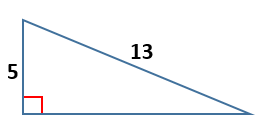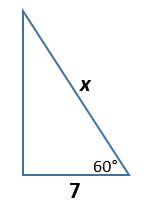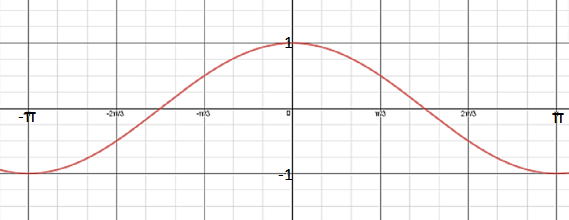# Geometry & Trigonometry Flashcards

Geometry & Trigonometry Flashcards
1/13 (missed) 0 0
Create Your Account To Continue Studying

As a member, you'll also get unlimited access to over 75,000 lessons in math, English, science, history, and more. Plus, get practice tests, quizzes, and personalized coaching to help you succeed.

Try it risk-free for 30 days. Cancel anytime.
###### Already registered? Login here for access
Calculate the length of the missing side.The missing side of the triangle has a length of 12.
Got it
List the three important steps to visualizing a geometry problem.

1. Gather the important information.

2. Draw a diagram of the problem.

3. Check your results.

Got it
Determine the length of the missing side.The missing side of the triangle (labeled x) has a length of 14.
Got it
State the ratio of the triangle sides that you would use to calculate the sine of an angle.
The sine is found by dividing the side opposite the angle by the hypotenuse of the right triangle.
Got it
State the ratio of the triangle sides that you would use to calculate the cosine of an angle.
The cosine is found by dividing the side adjacent to the angle by the hypotenuse of the right triangle.
Got it
Determine the volume of a cube that measures 2 inches along each side.
The volume of the cube would be 8 cubic inches.
Got it

### Ready to move on?

or choose a specific lesson: See all lessons in this chapter
13 cards in set

## Flashcard Content Overview

If you are looking at a right triangle, do you remember all of the relationships that exist between its sides and its angles? These flashcards will help you review the Pythagorean Theorem, so that you can calculate the third side of a right triangle if you know the other two sides. You will also get some practice with the trigonometric functions of sine and cosine which give you relationships between angles and sides. Additionally, there is some review of volume and setting up geometry word problems.

Front
Back
Determine the volume of a cube that measures 2 inches along each side.
The volume of the cube would be 8 cubic inches.
State the ratio of the triangle sides that you would use to calculate the cosine of an angle.
The cosine is found by dividing the side adjacent to the angle by the hypotenuse of the right triangle.
State the ratio of the triangle sides that you would use to calculate the sine of an angle.
The sine is found by dividing the side opposite the angle by the hypotenuse of the right triangle.
Determine the length of the missing side.The missing side of the triangle (labeled x) has a length of 14.
List the three important steps to visualizing a geometry problem.

1. Gather the important information.

2. Draw a diagram of the problem.

3. Check your results.

Calculate the length of the missing side.The missing side of the triangle has a length of 12.
Give the function shown in the graph.The function shown is f(x) = cos x.
A right triangle has a hypotenuse of 7 inches. Its shortest side measures 3 inches. Determine the length of the third side of this triangle.
The third side would have a length of the square root of 40 or approximately 6.32 inches.
Give the length of the shortest side of a right triangle that has two sides measuring 4 and 5.
The shortest side would have to measure 3.
A fire ladder is used to reach a window 24 feet off the ground and the base of the ladder is 10 feet away from the wall. Determine the length of the ladder.
The fire ladder must be 26 feet long.
A right triangle has two sides that measure 8 and 9 inches. Find the length of the hypotenuse of this triangle.
The hypotenuse of this triangle would measure the square root of 145 or approximately 12.04 inches.
Formula for the volume of a solid with uniform cross-sections
To find the volume of a solid with uniform cross-sections, you multiply the area of the cross-section by the height of the solid.
Pythagorean Theorem
a2 + b2 = c2 where c is the hypotenuse of the right triangle.

To unlock this flashcard set you must be a Study.com Member.